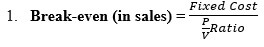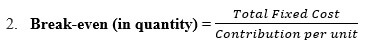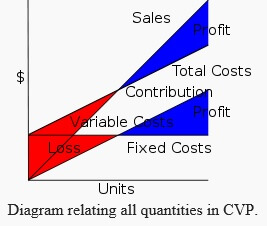# Cost Volume Profit Analysis Assignment Help

## Cost Volume Profit Analysis

A CVP Model is based on managerial economics and is a part of cost accounting. It takes vital decisions related to the short term functions of business. One of the important constraints of the CVP Analysis is the point where the total cost and total revenue are equal. At this point there is no profit or loss to the business. This is same as the Break-even Analysis. It works throughout on the BEP strategy. CVP Analysis has the same assumptions as that of Break-even point. It is just that the BEP lays down the initial steps and the CVP gives a detailed insight to the subject.

## The components of CVP Analysis are:• Level or volume of activity
• Unit selling prices
• Variable cost per unit
• Total fixed costs

### CVP assumes the following:

• Constant sales price;
• Constant variable cost per unit;
• Constant total fixed cost;
• Units sold equal units produced.
• Contribution stands for sales minus variable costs.

One of the main methods of calculating CVP is profit–volume ratio which is (contribution /sales)*100 ; this gives us profit–volume ratio. Therefore it gives us the profit added per unit of variable costs.

### Basic graph

The basic graph of CVP Analysis is also just like the Break-even analysis. The CVP is also a linear graph only because of its assumptions which remain constant and make the graph linear i.e. a straight line. During the formation of the graph the basic assumption is that whatever is produced is ultimately sold i.e. the no. of units produced = no. of units sold.

The basis of graph can be understood with the following explanation:

```In symbols,
TC = TFC+ V X X
TR = P X X
Where,

TC = Total costs
TFC = Total fixed costs
V = Unit variable cost (variable cost per unit)
X = Number of units
TR = S = Total revenue = Sales
P = (Unit) sales price
```

Profit is computed as TR-TC; it is a profit if positive, a loss if negative.

### Break down

Cost and sales can be further understood by breaking up Cost and Revenue further. The format shows the format of calculation of contribution and final profit.

ParticularsAmount
SalesXXX
Less: Variable Cost(XXX)
ContributionXXX
Less: Fixed Cost(XXX)
EBITXXXThe various formulae for analysis of break-even point are;where,

P/V Ratio = (Contribution/Sales) *100.3. Margin of Safety = Margin of safety = (current output - breakeven output)
Margin of safety shows the strength of the business. It shows where the business stands how safe its functions are. It shows if the business is above the Break-even point i.e. earning profit; or below the Break-even point i.e. incurring losses.

4. Margin of safety% = (current output - breakeven output)/current output × 100

### Analysis of Break-even Point:

The CVP Graph can be visualized as follows:This diagram shows the use of CVP Analysis. In the diagram it is clearly visible that one sale covers only the fixed costs and one covers both fixed costs as well as variable costs. The point of sales where only fixed costs are covered is called shut-down point whereas the point where the sales intersect with the total cost i.e. Fixed as well as variable; that point is called break-even point. Any sale beyond the break-even point will determine the profits for the firm.

#### Applications:

CVP Analysis helps in the computation and easy applicability of the Break- even analysis. It is a useful tool in ascertaining the best possible functions for the business and also evaluates the short term policies of the business.

Following are the fields where CVP Analysis is used:

1. Price Fixation
2. Accepting Special Order and Exploring Additional Markets
3. Profit Planning
4. Key Factors or Limiting Factor
5. Sales Mix Decisions
8. Suspension of Activities

#### Limitations:

1. It takes into consideration the cost side only. It does not study the sale variable on the products. This is a shortcoming as the cost does not influence the working of the business all alone.
2. This system assumes that the fixed costs remain constant which is not entirely true in the short run. All the costs tend to fluctuate in the short run.
3. It assumes whatever is produced gets sold which is not true in practical life. It assumes that the cost of production of every unit is the same which might not hold true.
4. Segregation of Fixed cost and variable cost is a daunting task.